Experiments with simulation of confinement of ions in a static electromagnetic field

11.17  Confinement of positive ions and electrons with a static electromagnetic field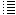Generating the particles all over the vacuum chamber with zero (or small) speed, injecting them and representing 1E9 or1E10 particles by only one

 Experiment 11.17.1.  SEM fusor with reversed polarities; particles generated with zero speed all over the vacuum chamber. 600 D+ ions and 600 electrons.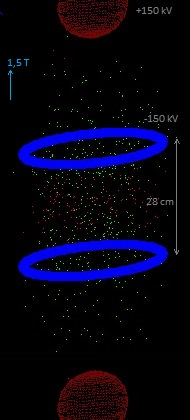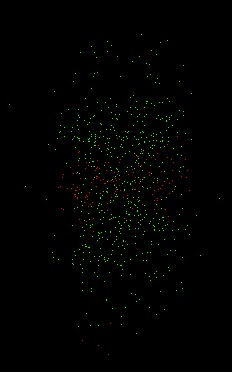Some D+ ions obtain speeds till 2,3E6 m/s (more or less in the centre region, see the screenshot). Many electrons disappeared (that were generated outside the region between the two blue rings). Also some D+ ions disappeared. By doing the same experiment but only generating 80 D+ ions and counting: almost all D+ stay confined, although some might collide with the blue rings (in the simulation they cannot colide with or enter into the charged rings and spheres, although it would be possible to program "something"). A part of the electrons stay confined between the blue rings and form a kind of negative charged cloud. Many electrons escape. It's a pity that the D+ ions do not move towards a point in the centre (like in a fusor). But they move up and down, stay confined, and ions with opposite speed could collide with each other. What is the mean speed of D2 molecules at 293 º K? ½ . m . v2  = 3/2 . k . T  (see: hyperphysics kinetic temperature ) k = Bolzmann constant = 1,38 E-23 J/K      ( k = R/Na,  R=8,31 Nm/mol, K    Na = 6,0 E23) m = massa D2 molecule =  2. 2.  1,67E-27 = 6,68E-27 kg v2 =  3/2 . 1,38 E-23 .293 / ( ½ . 6,68E-27 ) v  = 1,35 . 103 m/s Experiment 11.17.2.  SEM fusor with reversed polarities; particles generated with random speeds up to 2 . 103 m/s  all over the vacuum chamber. 100 D+ ions and 100 electrons.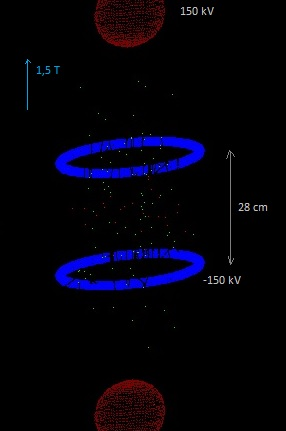Almost all D+ ions stay confined. Many electrons escaped; 23 electrons stay confined between the blue rings. Experiment 11.17.3.  SEM fusor with reversed polarities; particles generated with random speeds up to 2 . 103 m/s (more or less room temperature) all over the vacuum chamber. 100 D+ ions and 100 electrons.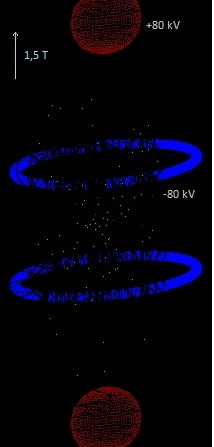Almost all D+ ions stay confined. Many electrons escaped; only 7 electrons stay confined between the blue rings. The D+ ions achieve quite high speeds, up till about 2,2E6 m/s. (see screenshot). The confined electrons have speeds between about 105 till 107 m/s (in the simulation). Conclusion: Suppose we have a vacuum chamber just between the red spheres and in between the blue rings, with a low density D2 gas at room temperature (293 K) , inside a strong magnetic field of 1,5 tesla. We apply then the +/- voltages of 80 kV (relative small compared with the +/- 200 kV of the other simulation experiments). The deuterium gas will be ionized (I suppose), and this will be similar as generating D+ ions and electrons with random speeds up to 2 . 103 m/s as in experiment 11.17.3. The D+ ions stay confined in the simulation and obtain speeds up to 2,2 106 m/s. (for fusion a mean speed of about 2.106 m/s is needed). The electrons stay less confined; perhaps they could be injected. Experiment 11.17.4.  SEM fusor with reversed polarities. 100 D+ ions generated : vid=3E3 m/s; ve:=vid*2;  {vid is chosen in the menu of the program, 3E3 m/s} hydrogen[i].vx:=0 + (-0.5 + random)*ve/1000;  {hydrogen=deuterium} hydrogen[i].vy:=0 +( - 0.5 + random)*ve/1000; {random -> 0 <= X < 1 } => a small initial random speed in the x and y direction hydrogen[i].vz:=0 +( - 0.5 + random)*ve/1000 + vid; => initial speed in the z direction = 3E3 m/s (more or less the "room temperature speed")  + a small random speed. hydrogen[i].x:=0.5+ ( - 0.5 + random) /10000; hydrogen[i].y:=0.5+ ( - 0.5 + random) /10000; hydrogen[i].z:=0.10 + ( - 0.5 + random) /10000; {so they start not in exactly the same point} Coordinate system The centre of the red sphere under is at ( x=0,5, y=0,5 , z=0,00) and the top of this red sphere is at (x=0,5, y=0,5 , z=0,08). The D+ ions are generated at 2 cm from the top of the red sphere under. 110 electrons generated: ve:=2*vie;  vie = 1E4 m/s (chosen in the menu of the program) electron[i].vx:=0 + (-0.5 + random)*ve/1000; electron[i].vy:=0 + (-0.5 + random)*ve/1000; electron[i].vz:=0 + (-0.5 + random)*ve/1000+vie; => a small initial random speed in the x and y direction electron[i].x:=0.52+ ( - 0.5 + random) /10000; electron[i].y:=0.52+ ( - 0.5 + random) /10000; electron[i].z:=0.35+ ( - 0.5 + random) /10000; {so they start not in exactly the same point} The centre of the blue ring under is at ( x=0,5, y=0,5 , z=0,30) The electrons are generated 5 cm above the centre of the blue ring under with a vertical speed of 1E4 m/s. ½mv2 = q.ΔV  => ΔV = 0,5 . 9,1E-31.(1E4)2  /1,6E-19 = 2,84E-4 volt  (m=massa electron; v=speed electron, q=charge electrons; ΔV = voltage difference needed to accelarate the electrons) This is only a small voltage difference...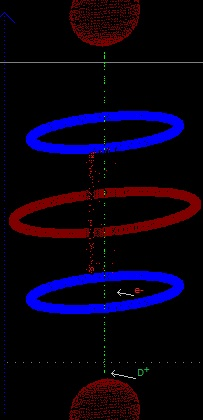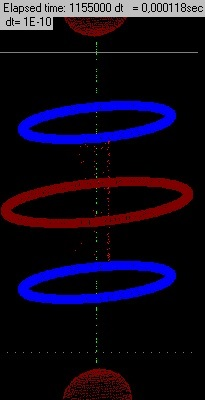Without the middle ring the electrons escape.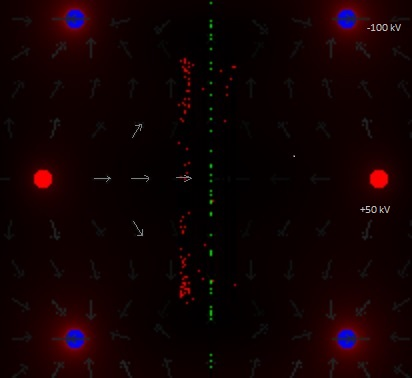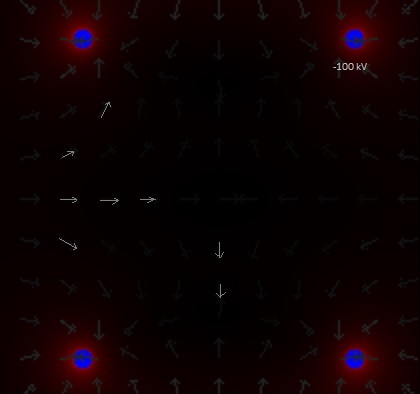Above the electric field. In the second image the middle ring has been taken out. The direction of the electric field does not change a  lot, but the strength yes, so all the electrons escaped. When the blue rings are charged with -200 kV, new generated electrons stay confined. The D+ ions feel in the centre region a sideward electric force towards the vertical centre line, which is favourable. After all we want them to collide and to fuse in the centre region. At the contrary, the electrons feel here an electric force outwards. Will they loose their speed because of Bremsstrahlung and then escape sidewards? But if the D+ agglomerate in the centre, their positive charge will attract the electrons. The potential in the point were the electrons are injected/generated is about -32E3 volt (the program calculates the potential in the y-z plane (x=0,5 m) when clicked with the mouse in a point, The energy needed to get one electron there, from outside the SEM (from infinity): E (1 e-) = q.ΔV = 1,6E-19 . -32E3  =  5,12E-15 J Suppose we filled our vacuum chamber of 0,16 m3 with 3E-2 torr D2 gas, which corresponds with 1,1 mg D2 gas, which corresponds with   96,6E6 J = 2,4 ltr diesel (see Droom11.16.htm )  (1 gram deuterium = 8,78E10 J; 1 J = 2,6E-8 ltr diesel  energy conversion  conversions ) 1,1 mg D2 gas = 2,63E-4 mol   (1 mol = 6,022 E23 molecules D2) = 2,63E-4 . . 6,022E23 . 2 = 3,17E20 D+ ions. The same amount of electrons => E ( 3,17E20e-) = 3,17E20 . 5,12E-15 = 1,62E6 J = 0,042 ltr diesel  =>  it does not cost a lot of energy to bring the electrons into the SEM (the amount of electrons that corresponds with the amount of D+ ions , if all D2 gas is ionized).   The potential in the point were the D+ are injected/generated is about 45E3 volt. The energy needed to get one D+ there, from outside the SEM (from infinity): E (1 D+) = q.ΔV = 1,6E-19 . 45E3  =  7,2E-15 J To bring all D+ ions inside the SEM (the same calculations as above, with the electrons), would need  3,17E20 . 7,2 E-15  = 2,2E6 J = 0,058 ltr diesel. => this neither costs a lot of energy J Experiment 11.17.5.  SEM fusor with reversed polarities.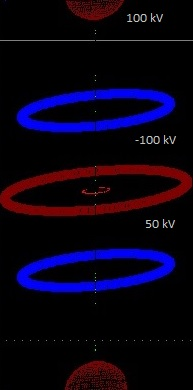Same as experiment 11.7.4, only the speed of the electrons is (slowly) made smaller after they have been generated. In reality the electrons will slow down (in a matter of seconds) because of Bremsstralung. In the simulation it is going a lot faster. in  procedure Move2_particle(var particle: Tparticle); if t=1 then {for electrons} begin vx:=vx*0.999; vy:=vy*0.999; vz:=vz*0.999; {so the speed of the electrons slowly decreases } end; particle.vx:=vx; {the new position of particle in the x-direction after dt sec} particle.vy:=vy; particle.vz:=vz; Curious that the electrons form more or less a circle. The distance of the electrons to the vertical centre line slowly increases in the simulation: Δr / Δt = 100 m/s  (r= distance to vertical centre line, t = time ) In 0,001 s the distance r increase about 10 cm.  Note: this is the maximum distance, not the mean distance of all electrons. But let's suppose that we have to replace all electrons in 0,001 s. 1,1 mg D2 gas (see exp. 11.17.4.) corresponds with 3,17E20 D+ ions. = 3,17E20 e-. 3,17E20 e- /0,001 s = 3,17E20 . 1,6E-19 coulomb / 0,001 s  =  50720 C/s = 50,72 kA  which is huge and not acceptable! We will not have a efficient fusion reactor is this way. But the electrons will also be atracted by the D+ ions that cluster in the centre, I suppose. It´s a pity we cannot simulate 3,17E20 particles..   However, let's calculate the mean distance of all electrons in the program and see what happens.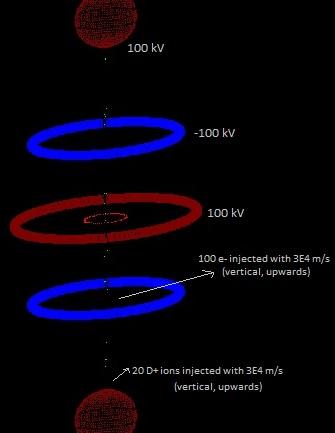The mean distance of the electrons to the vertical centre line is also increasing. Δr / Δt = 188 m/s  (see the screenshot ; r= distance to vertical centre line, t = time ) The same experiment, but the reduction of the speed is slower: if t=1 then  {electron} begin vx:=vx*0.99999; vy:=vy*0.99999; vz:=vz*0.99999; end; particle.vx:=vx; {the new position of particle in the x-direction after dt sec} particle.vy:=vy; particle.vz:=vz; Screenshot Δr / Δt = 167m/s  (see the screenshot) Experiment 11.17.6.  SEM fusor with reversed polarities.The magnetic field is as a magnetic bottle, generated by the current loops. In Procedure Move2_particle(var particle: Tparticle); if t=1 then begin vx:=vx*0.99999; vy:=vy*0.99999; vz:=vz*0.99999; end; {the speed of the electrons is slowly made smaller, like Bremsstrahlung, but a lot faster than in reality } if vx>1E7 then vx:=1E7; if vy>1E7 then vy:=1E7; if vz>1E7 then vz:=1E7; if vx<-1E7 then vx:=-1E7; if vy<-1E7 then vy:=-1E7; if vz<-1E7 then vz:=-1E7; {to avoid speeds too high (even > 3E8..)} In Procedure Generate_Electron electron[i].m:=me*3E9; electron[i].q:=-qe*3E9; {3E20 is the amount of D+ in the vacuum chamber if p=3E-2 torr} In Procedure Generate_Hydrogen hydrogen[i].m:=mp*3E9 hydrogen[i].q:=qe*3E9;  {actually is deuterium, qe is charge electron} We cannot simulate 3E9 particles. But now we group this amount of particles in one. Because both the mass and the charge increase with the same amount, the acceleration of this "group of particles" will be the same as the one of only one particle. What also happens now is that the "group of particles" interact amongst themselves, what does not happen if we generate normal particles (because we can only generate a couple of hundred, which are infinite small and at very large distances of each other in the vacuum chamber). Probably because we do not take into account relativistic effects and because the calculation time dt is perhaps to big (causing sometimes  "group of particles" coming too close, producing too big Coulomb forces ~ 1/r ), some "group of particles" obtained  too high speeds (even larger than 3E8 m/s). So I included the program a algorithm that avoid speeds higher than 1E7 m/s. In the screenshot can be seen that the mayority of the electrons are confined in the centre region, although they have spread out in the x and y direction. The D+ ions reach quite high speeds, many more than 2E7 m/s ( speed necessary fo D-D  fusion ) See  second screenshot . The same experiment, but now: electron[i].m:=me*3E10; electron[i].q:=-qe*3E10; hydrogen[i].m:=mp*3E10 hydrogen[i].q:=qe*3E10;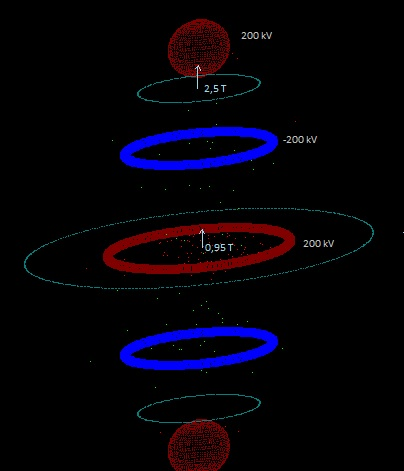The particles spread out sidewards, probably due to the mutual interactions. The same experiment, but changed: {the speed of the electrons is made smaller, but the process is slower} if t=1 then begin vx:=vx*0.9999999; vy:=vy*0.9999999; vz:=vz*0.9999999; end; Screenshot Experiment 11.17.7. SEM fusor with reversed polarities.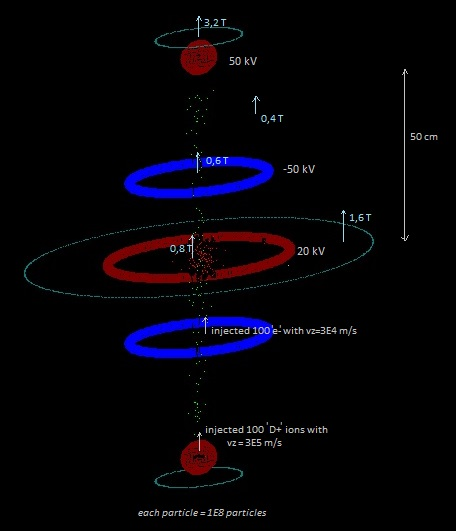Algoritme with speed limit < 1E7 for all particles. Each particle has a mass and charge x 1E8 (is a kind of group of  1E8 particles represented by one). The D+ are injected with a speed of 3E5 m/s ->  ½ mv2 =  ΔV .q  -> ΔV = 0,5 . 2 . 1,67E-27 . (3E5)2 /(1,6E-19) = 939 V The e- are injected with a speed of 3E4 m/s  ->  ΔV = 0,5 . 9,1E-31 . (3E4)2 /(1,6E-19) = 2,6 mV Time lapse = 2,38E-5 s The magnetic field is quite strong; the voltages are not so high (compared with the former experiments). hydrogen[i].vx:=0 + (-0.5 + random)*ve/1000; {so the maximum speed is vid} hydrogen[i].vy:=0 +( - 0.5 + random)*ve/1000; {random -> 0 <= X < 1 } hydrogen[i].vz:=0 +( - 0.5 + random)*ve/1000 + vid; hydrogen[i].x:=0.5+ ( - 0.5 + random) /100{4} {20}; {so they start not in exactly the same point} hydrogen[i].y:=0.5+ ( - 0.5 + random) /100{4}{20}; hydrogen[i].z:=0.10 + ( - 0.5 + random) /100{20}; {1.5) } This could be the best design: SEM fusor with voltages +/- 50 kV , with the configuration as above, and a magnetic field somewhat in the form of a magnetic bottle produced by a high temperature superconductor (HTS). The same experiment (same dimensions, voltages, magnetic field etc.) , but now every particle is a "real" electron and deuterium ion (with charge 1,6E-19 C and mass 1,67E-27 and 9,1E-31 kg).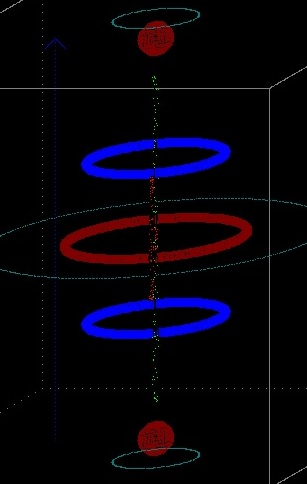Time lapse = 2,82E-5 s No speed limit. Experiment 11.17.8.  Various experiments SEM fusor with reversed polarities.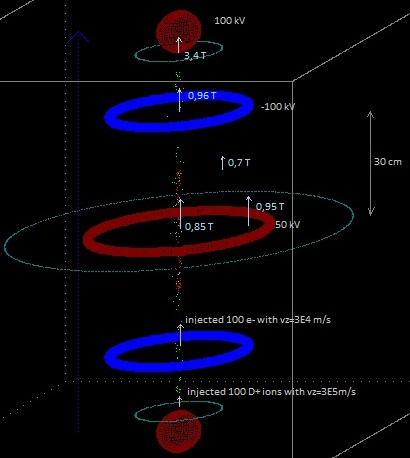Normal particles (electrons and D+ ions). No speed limitation, speed is not decreased. Some values of the magnetic field are indicated. Simulation about two days running. During about one day the total energy of the particles (kinetic + potential) stayed constant (an indication that the program/leap frog integration is working well) , and the maximum radius of the D+ and electrons also stayed constant. Then one D+ possibly interacted (collided) with another particle  and got a somewhat larger radius. See the screenshot. The deuterium ions reach speeds of almost 2E6 m/s. To inject the D+ ions:  ½ mv2 =  ΔV .q  -> ΔV = 0,5 .2. 1,67E-27 . (3E5)2 /(1,6E-19) = 939 V The same experiment but now with a homogeneous magnetic field of 1,5 tesla:Similar, but with a little bit different voltages, magnetic field strenght and dimensions: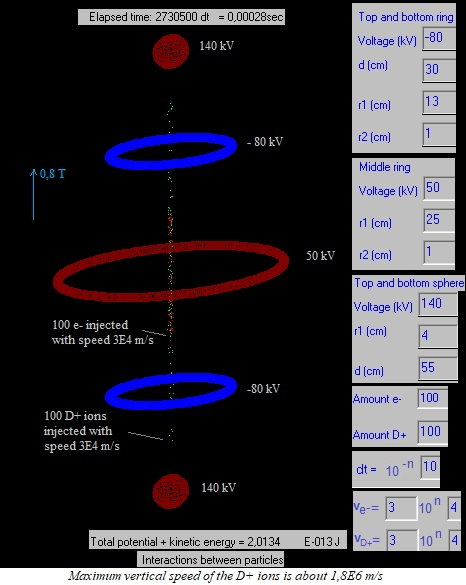Injecting the D+ ions with higher speed does not result in a lot higher vertical speed. But increasing the negative voltage of the blue rings yes: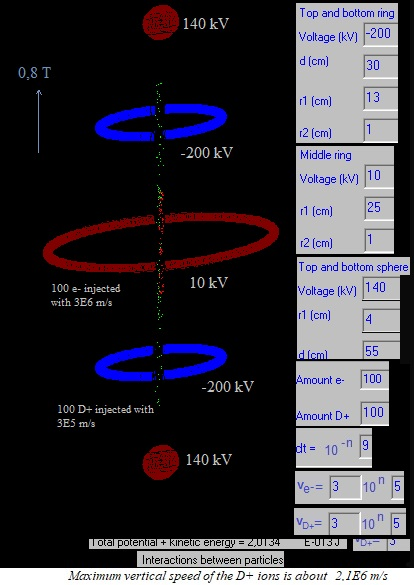Now with only two rings at - 80 kV and a magnetic field in the form of a magnetic bottle: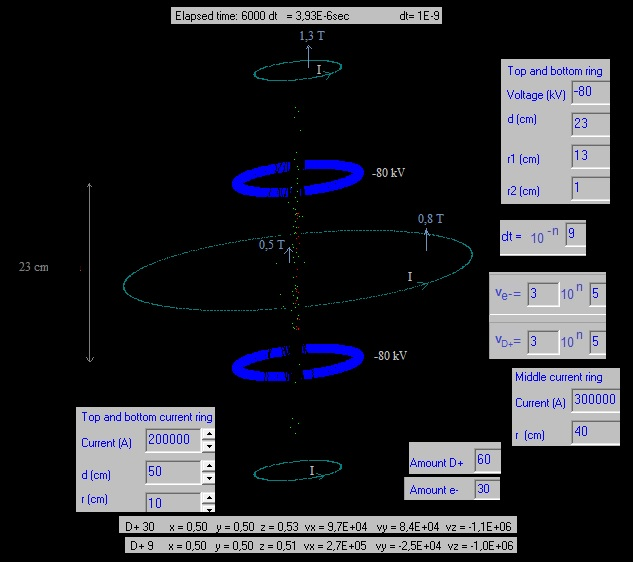The configuration with only two blue rings does not confine very well the particles. After some will escape after some time.   In the following experiment the voltages and the magnetic field are relatively low: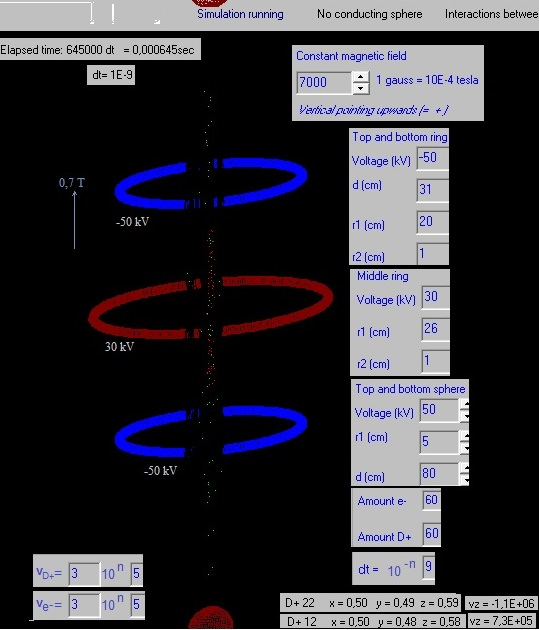The particles stay still confined, in the simulation. After one night running the simulation: The particles stayed confined, the total enery stayed constant (indicating that the simulation program is correct), and the maximum radius of the particles hardly changed. If we only inject deuterium ions, how many we could inject? 1,1 mg D2 gas ≈ 96,6 E6 J ≈ 2,4 ltr diesel,  see energy calculation . All ions are positive, and accumulate then a charge:  3,17E20 . 1,6E-19 = 50,7 coulomb. Imagine that this charge is divided into two charges, at a distance of 1 mtr of each other. The force between these two charge would be: F=9E9 . 25. 25 / 1 = 5,6 E12 N = 5,6 E11 kgf = 5,6 E 8 tonne-force , so imposible. If we would inject only 1,1 . 1E-3 mg (a thousend times less), this would correspond with an energy (if all D+ ions would fuse) of 2,4E-3 ltr diesel (about 1 gram diesel), but the force between two parts of the charge (if divided in two and at 1 mtr distance) would be 5,6 E6 N = 5,6 E5 kfg = 560 tonne-force, so still huge... Conclusion of this rough calculation: by injecting only D+ ions and trap them as in a Penning Trap, we could only inject very small amounts and if fusion would occur, only get very little bit of energy release. Experiment 11.17.9 If we inject and confine boron ions B+ , hydrogen ions H+ and electrons, perhaps we achieve the reaction: 115B + 11H  -> 3 42He  + 8,68 MeV An advantage of this fusion reaction with boron is that it not produces neutrons, which are dangerous and noxious. See also: Wikipedia.org/wiki/Aneutronic_fusion In the computer simulation we make the mass of the D ions:  mp=11*1.6726E-27, so they become B+ ions (with charge + 1,6E16 C)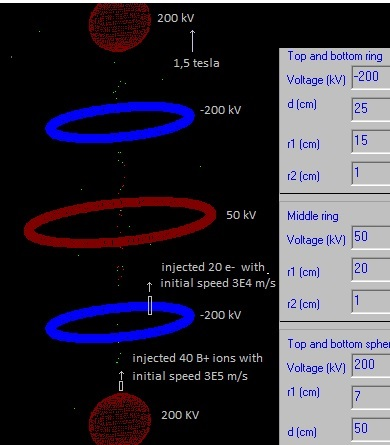Result: the B+ have about 6 times more mass than the D+ ions, so as can been seen, they are less confined sidewards by the magnetic field. Hydrogen H+ I did not inject, but as can be understood easily, because they are lighter, they will be better confined. If a real experiment would be carried out, I think it should be done first with deuterium. If fusion occurs in the SEM fusor, then this could be detected by measuring the neutrons that are created. Later on perhaps experiments could be done with boron and hydrogen. To confine well the boron ions, quite probably the magnetic field must be stronger. It seems not to be possible (or difficult) to make B2+, B3+ etc. Experiment 11.17.10 Generating the deuterium ions and the electrons randomly, more or less in the vacuum chamber. Result: A part of the D+ ions and of the electrons stay confined. Some D+ ions obtain quite high speeds > 1E6 m/s. What happens if 80 % of the D+ collide with the blue rings and 80 % of the electrons colide with the red spheres? Suppose we filled our vacuum chamber of 0,16 m3 with 3E-2 torr D2 gas, which corresponds with 1,1 mg D2 gas, which corresponds with   96,6E6 J = 2,4 ltr diesel (see Droom11.16.htm )  (1 gram deuterium = 8,78E10 J; 1 J = 2,6E-8 ltr diesel  energy conversion  conversions ) 1,1 mg D2 gas = 2,63E-4 mol   (1 mol = 6,022 E23 molecules D2) = 2,63E-4  . 6,022E23 . 2 = 3,17E20 D+ ions. 80 % of this is: 2,5E20 D+ ions ->  * 1,6E-19 C = 40 C. To keep the blue rings at -150 kV we need to supply a negative charge of -40 C (electrons). The work (labour) this takes is: 150 kV . 40 C = 6000 kJ = 0,16 ltr diesel. The same for the lost electrons. If 20 % of the deuterium ions fuses we get 20% x 2,4 ltr diesel = 0,48 ltr diesel. (converted this in ltr diesel to get some more feeling with it) To keep the spheres and rings charged we need 2x0,16 = 0,32 ltr diesel. This simple calculation demonstrates that this design will probably not be very effective. As mentioned before, better is to inject the deuterium ions and the electrons. But this is a little bit more complicated to realize. A first experiment could be done without injecting the particles; just filling the vacuum chamber with deuterium gas. If neutrons are then detected, this would already be a great discovery and prove that fusion is possible. However, if we have enough budget, we could perhaps immediately realize a vacuum chamber with the possibility to injects the ions and the electrons.31 January 2018 (12 March 2018)      by  Rinze Joustra        www.valgetal.com